for HP Prime
An implementation of
Lambert W function and exponent of Lambert W function
for HP Prime

Oct. 25 2020
Takayuki HOSODA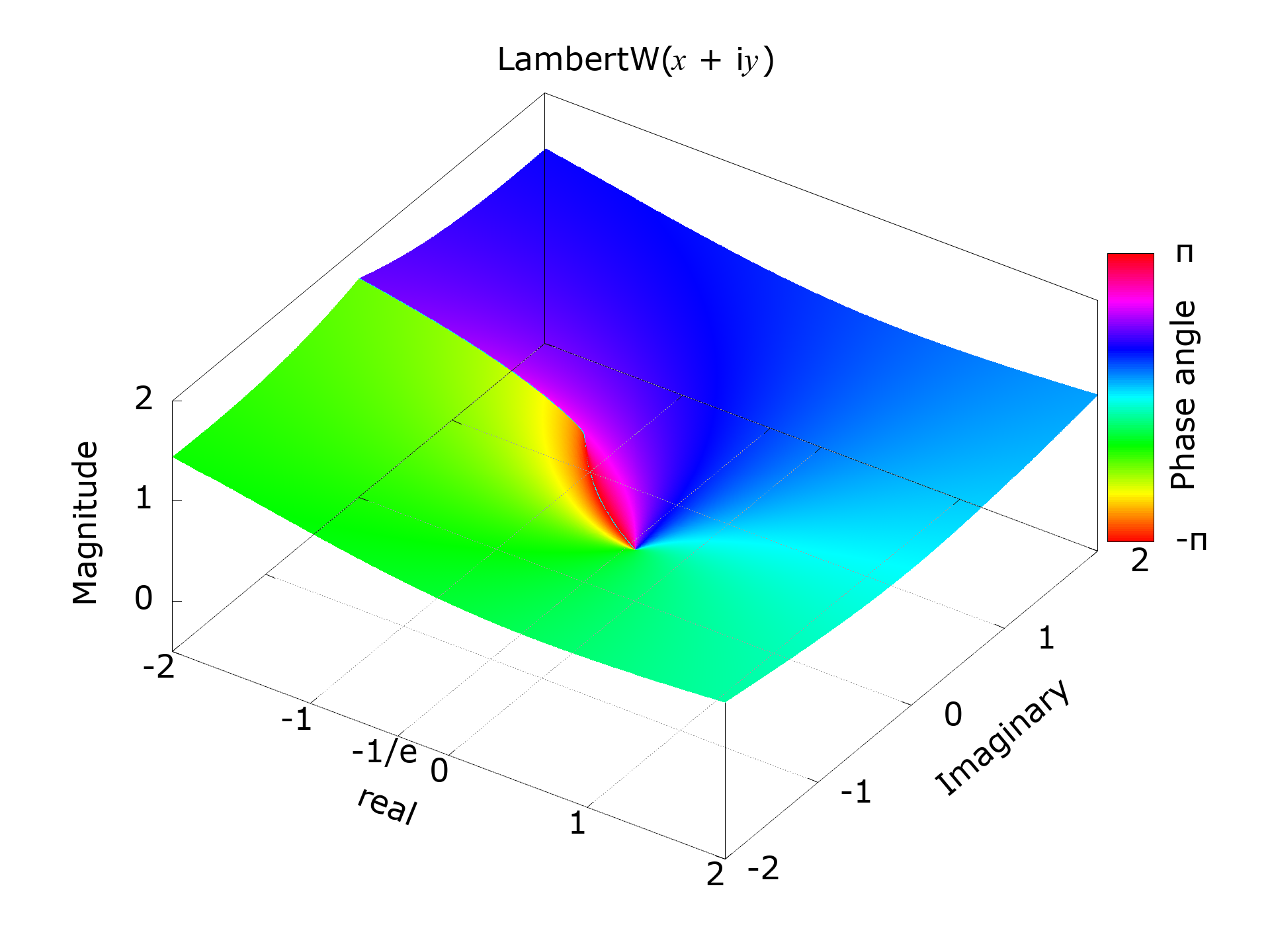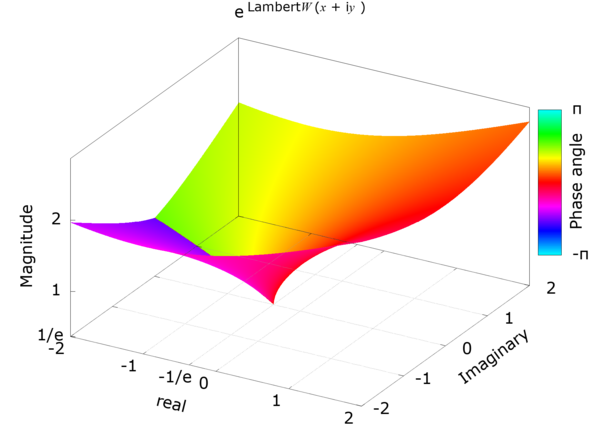From the relation below, the Lambert W can be calculated by using eW — the exponent of Lambert W function.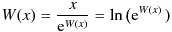eW0 — Exponent of the principal branch of the Lambert W function

eW0(x) — the exponent of the principal branch of the Lambert W function W0(x) — can be calculated by using Newton-Raphson method,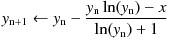or Halley's method shown below.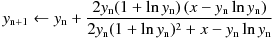When finding eW using an iterative method such as Newton's Rapson method, giving a bad initial value, especially when it is very close to the singularity x = -1 / e, can be terrible in terms of convergence. Therefore, it is desirable that the initial value asymptotically approaches eW at the singularity. For that reason, I chose the following formula as an approximate expression that gives the initial value. This equation replaces the coefficient 1 / 3 of the third term of the Piaseux series expansion of e W0 at x = -1 / e with 0.3 and ignores the fourth and subsequent terms.

Initial approximation :Series expansion of eW at z = -1 / e (Puiseux series) :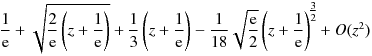Instead of the coefficient of 0.3 in the formula above, it can be (e - √2 - 1) which make it zero at x = 0,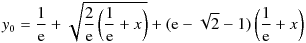or "1 / 3" from Puiseux series, but 0.3 is quite good enough for this purpose.

It's nice to be able to handle complex numbers easily with HP Prime, and thanks to sqrt √ in this equation, HP Prime can automatically get the complex y0 from x less than -1 / e.
Note that, complex output from real input must be allowed by selecting the check box of 'Complex' in 'Home Setting'.

 eW (for HP Prime) eW(x) returns the requested exponent of the principal branch of the Lambert W function. Recurrence formula (Halleys method) :Initial approximation :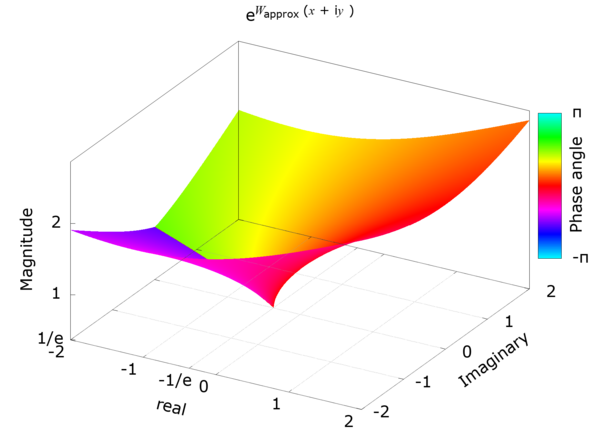#CAS //eW : exponent of the Lambert W0 function //Rev.1.52 (Dec. 4, 2020) (c) Takayuki HOSODA (aka Lyuka) // http://www.finetune.co.jp/~lyuka/technote/lambertw/ //Acknowledgments: Thanks to Albert Chan for his informative suggestions. eW(x):= BEGIN LOCAL y, p, s, t, u, v; LOCAL q, r, m; HComplex := 1; // Enable complex result from a real input. r := 1.0 / e; q := e - sqrt(2) - 1; s := x + r; IF s == 0 THEN return r; END; m := MAXREAL / 1024.0; IF abs(RE(x)) <= m AND abs(IM(x)) <= m THEN m := 1.0; y := r + sqrt(2.0 * r * s) + q * s; // approximation near x=-1/e ELSE m := 1.0 / 1024.0; x := x * m; s := x * m; y := r * m + sqrt(r * (s * 64.0)) + q * s; y := y * 1024.0; END; r := MAXREAL; REPEAT q := r; p := y; t := ln(y); v := 1 + t; s := 0.5 * (x * inv(y) - t * m) * inv(v) + v * m; //s <> 0, y <> 0, v <> 0 t := (x - y * (t * m)); y := y + t * inv(s); // Halley's method r := cabs(y - p); // correction radius UNTIL 0 == r OR q <= r; // convergence check return p; END; // This is a workaround for the dynamic range problem of complex division and abs() in CAS. // Conforms to HP Prime software version 2.1.14433 (2020 01 21). // cabs(z) -- returns the magnitude of the complex number z. cabs(z):= BEGIN LOCAL x, y, t, s; x := RE(z); y := IM(z); IF x < 0 THEN x := -x; END; IF y < 0 THEN y := -y; END; IF x == 0 THEN return y; END; IF y == 0 THEN return x; END; IF (y > x) THEN t := x; x := y; y := t; END; t := x - y; IF t > y THEN t := x / y; t := t + sqrt(1 + t * t); ELSE t := t / y; s := t * (t + 2); t := t + s / (sqrt(2) + sqrt(2 + s)); t := t + sqrt(2) + 1; END; return(x + y / t); END; #END 
Calculation results by WolframAlpha
• eW0(0) = 1
• eW0(1) ≈ 1.7632228343518967102252017769517070804360179866674736345704569055…
• eW0(ln(4)) = 2
• eW0(e) = e ≈ 2.7182818284590452353602874713526624977572470936999595749669676277…
• eW0(-1 / e) = 1 / e ≈ 0.3678794411714423215955237701614608674458111310317678345078368016…
• eW0(-1) ≈ 0.168376379087222910557029040196419798877374748292541336164446057… + i0.707754188784727616472845893076516239493840814237501557178104593…
• eW0(i) ≈ 1.21953141590463829076184958147631568018373928971052494273419018… + i0.792760480536266149513645901104205400304007958310758367947600535…
• eW0(1 + i2) ≈ 1.963022024795710615403657609352963399096103612439429976312270215… + i1.15790481862049460355866218250643368
• eW0(10) ≈ 5.7289255653869415083948334462695428583083011245188679977745329741…
• eW0(-10) ≈ -2.12160672801229585881839882039576808888691299603035504200805812… + i3.31438991652408887334308129325434704440288406766670451189320153…
• eW0(-i10) ≈ 2.722012666153399540620643911105461239034258583367698609451500201… ± i4.400126278029247932336458566649073775948416746853190590864610860…
• eW0(6 ± i8) ≈ 4.581959803814930785295395946842850387269808607228525308526722722… ± i3.089581433065026125734955412497412095982140159922896033902530108…
• eW0(8 ± i6) ≈ 5.162649626180324381723076303384526992159696714000299900472215322… ± i2.246055160453432964730517841645464483384472219052729726318907612…
• eW0(-8 ± i6) ≈ -0.40287723071326398891738198970518073669526101284395016165250318… ± i4.4530541067931410150849587479943346358241174711343684763480875…
• eW0(4 ± i3) ≈ 3.440104795843841636717760174891181763115768937098148854134632668… ± i1.327847267904875186994898739995810436465745575932480564120461721…
• eW0(3 ± i3) ≈ 3.009935716266451997748821597982744858525370046513717446847925057… ± i1.404458841055276492517218550954715584649647667572241839122054959…
• eW0(-3 ± i3) ≈ 0.481723888790768522809774752588540237351791197566637333002964266… ± i2.49933395776368232702661332288074194685329839029734865982822650…
• eW0(1e10 ± i1e10) ≈ 4.5756525984523291709515528101992994524165186338008534797672…× 10^9 ± i4.2804011082032989234103624115040439943310083779209001154467… × 10^9
• eW0(-1e10 ± i1e10) ≈ -3.95064930172318352041149250229341776540460561110958026114… × 10^9 ± i4.82903318856575595764115005895858802258618874972915085737… × 10^9
• eW0(1e150 ± i1e150) ≈ 2.94875501007892891390709858859972676434413169993120981577… ± i2.93519921644554515646774453247481152191756343715085628759... ~ 10^147 i

W0 — The principal branch of the Lambert W function

It is necessary to consider the singularity, but since eW does not become zero, it was relatively easy to find eW by Newton-Raphson method or Harrey's method. However, when trying to find W0 - Lambert's W of the primary branch by such an iterative method, since W0 becomes zero when x is zero, so it is necessary to consider zero as well as the singularity. In other words, it is strongly desired that the approximation formula of the initial value of the iterative method has an asymptotic to zero error at the singular point and zero. Also, since W0 is, from the singularity, a monotonically increasing function, its approximation must also be a monotonically increasing function. To meet these requirements, the initial value is calculated using the following approximation function.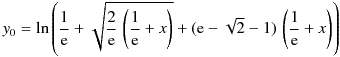Since W0 has a large derivative around the singularity, Newton's method can cause catastrophic behavior near the singularity if the initial approximation is poor or due to computational errors. Therefore, we will use the Halley method or Householder's method of order 4, which is more stable than the Newton method, to obtain W0.

In addition to the Halley's method of cubic convergence, the Householder's method of order 4 can also be used to calculate W0. However, special care is required because the calculation is complicated and it is easy for digits to overflow during the calculation. Householder's method was only a few percent faster than Halley's method for calculating up to 16 decimal places.

Newton-Raphson method :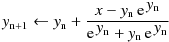Halley's method :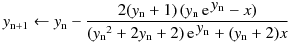Householder's method of order 4 :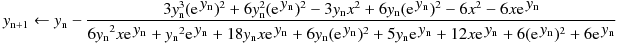The convergence test by Urabe's theorem is used to determine the convergence. It is a method to judge that the calculation limit has been reached and terminate the iteration when the correction value does not change or increases.

W0 calculation using Halley's method
 LW0C (for HP Prime CAS) LW0C(x) returns the requested value of the principal branch of the Lambert W function. Recurrence formula (Halley's method) :Initial approximation :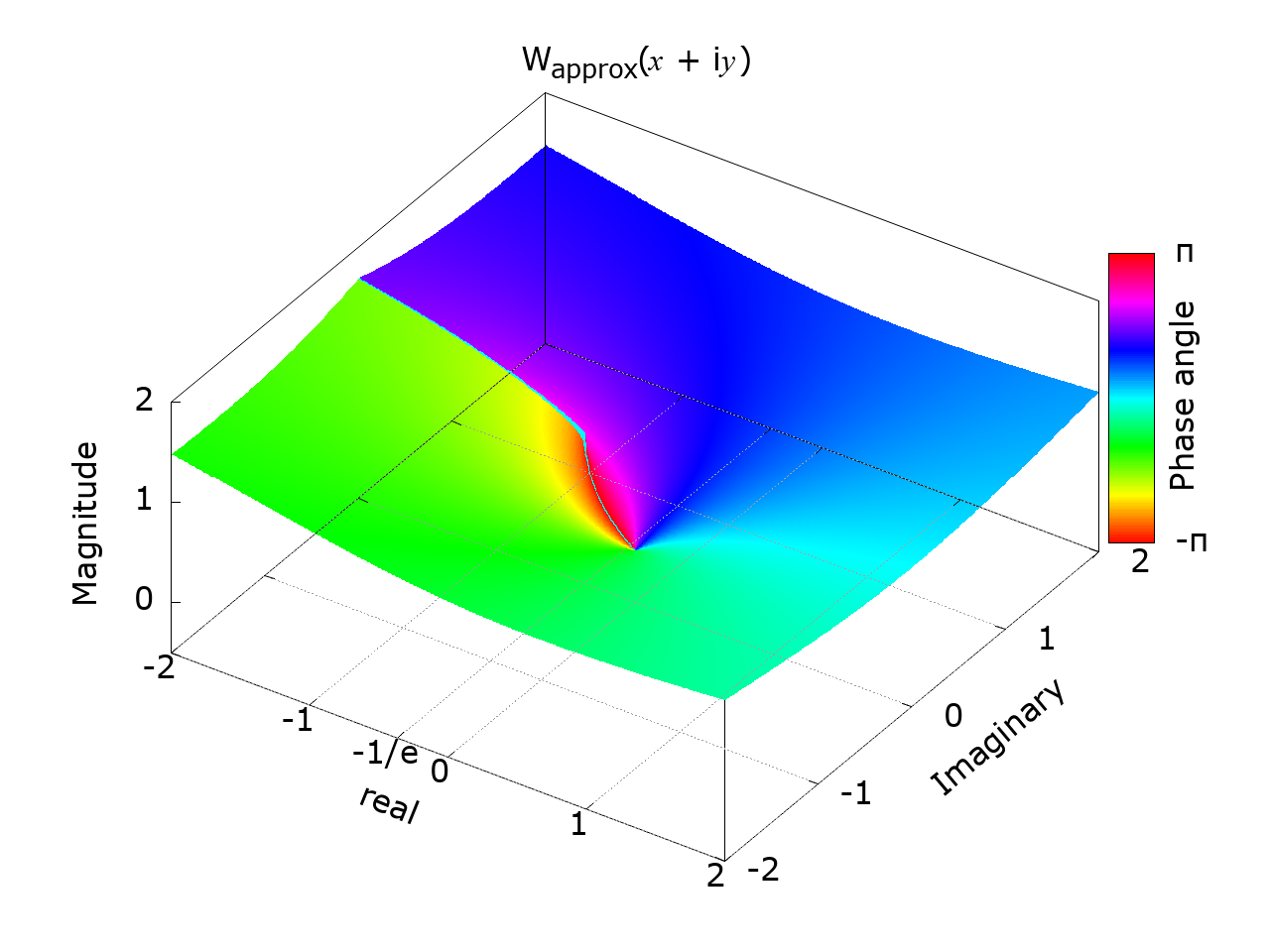#CAS //LW0C - Principal branch of the Lambert W function //Rev.1.52 (Dec. 4, 2020) (c) Takayuki HOSODA (aka Lyuka) //Acknowledgments: Thanks to Albert Chan for his informative suggestions. LW0C(x):= BEGIN LOCAL y, p, s, t; LOCAL q, r, m; HComplex := 1; // Enable complex result from a real input. r := 1.0 / e; q := e - sqrt(2.0) - 1.0; m := MAXREAL / 1024.0; IF abs(RE(x)) <= m AND abs(IM(x)) <= m THEN m := 1.0; s := x + r; IF s == 0 THEN return -1; END; y := ln(r + sqrt(2.0 * r * s) + q * s); // approximation near x=-1/e ELSE m := 1.0 / 1024.0; x := x * m; s := x * m; y := ln(r * m + sqrt(r * (s * 64.0)) + q * s); y := y + 6.931471805599453094; // + ln(2) * 10 END; r := MAXREAL; //1.79769313486e308 REPEAT q := r; p := y; t := exp(y) * m; s := y * t - x; t := (0.5 * y) * inv(y + 1.0) * s + t + x; // t <> 0 y := y - s * inv(t); // Halley's method r := abs(y - p); // correction radius; UNTIL 0 == r OR q <= r; // convergence check return p; END; #END 

Calculation example of LW0C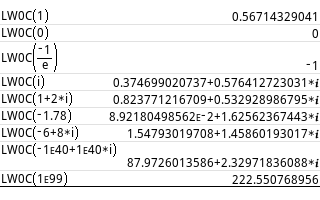Calculation results by WolframAlpha
• W0(0) = 0
• W0(1) ≈ 0.5671432904097838729999686622103555497538157871865125081351310792…
• W0(ln(4)) = ln(2) ≈ 0.6931471805599453094172321214581765680755001343602552541206800094…
• W0(e) = 1
• W0(-1 / e) = -1
• W0(-0.36) ≈ -0.806084315970817778285521361620992001997459968346671301630…
• W0(-1) ≈ -0.31813150520476413531265425158766451720351761387139986692237860… + i1.3372357014306894089011621431937106125395021384605124188763127…
• W0(i) ≈ 0.374699020737117493605978428759720807512802175326782642557502432… + i0.576412723031435283148289239887068476278099011222168280566265741…
• W0(1 ± i2) ≈0.823771216709230498962714234680902867860235005053071922240368278… ± i0.532928986795441605088201422572330085339330393308596795767937866…
• W0(-1.78) ≈ 0.089218049856209317716109060765294251305387407793167833503833064… + i1.6256236744277681331520891017303249073577948841857860157186500…
• W0(-6 ± i8) ≈ 1.547930197079635814768631631342423213473126810818780368642414024… ± i1.458601930168348176634851154910250705430582638721325124179334991…
• W0(1e99) ≈ 222.55076895575017934955228965217449610853244528249943629448650570…
• W0(-1e40 ± i1e40) ≈ 87.97260135857290602332406004222549277677935447060989442026705176… ± i2.329718360883123170160790314772387815611852082396629087352292852…
• W0(1e305) ≈ 695.74347234500662968414578760461819217555667677555452851562125354…
• W0(1e305 ± i1e305) ≈ 696.089548005772131650126785445013705743509108054188263218466334… ± i0.784271481992182658322800157708303004261113225909172777370356203
W0 calculation using Householder's method of order 4
W0 by Using Householder's method of order 4
 LW0H (for HP Prime) LW0H(x) returns the requested value of the principal branch of the Lambert W function. Recurrence formula (Householder's method of order 4) :Initial approximation :#CAS //LW0H - Principal branch of the Lambert W function //Rev.1.46 (Nov. 28, 2020) (c) Takayuki HOSODA (aka Lyuka) //Acknowledgments: Thanks to Albert Chan for his informative suggestions. LW0H(x):= BEGIN LOCAL y, p, s, t, u, v; LOCAL q, r; HComplex := 1; // Enable complex result from a real input. r := sqrt(MAXREAL) / 1e4; // Limit x range IF abs(RE(x)) > r OR abs(IM(x)) > r THEN return "LW0H: x out of range"; END; r := 1.0 / e; s := x + r; IF s == 0 THEN return -1; END; q := e - sqrt(2.0) - 1.0; y := 1.0 * ln(r + sqrt(2.0 * r * s) + q * s); // approximation near x=-1/e r := MAXREAL; REPEAT q := r; p := y; s := exp(y); t := s + x; u := y * s; v := u - x; v := (6.0 * ((y + 1.0) * u * x + t * (t + u)) + v * (3.0 * (u + x) + y * v)); IF (0 == v) THEN break; END; t := 3.0 * (u - x) * ((u + x) * (y + 2.0) + 2.0 * s); y := y - t * inv(v); // Householder's method of order 4 r := abs(y - p); // correction radius UNTIL 0 == r OR q <= r; // convergence check by Urabe's theorem return p; END; #END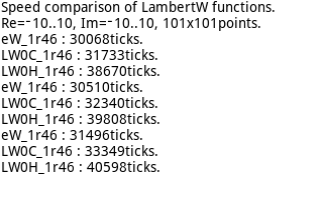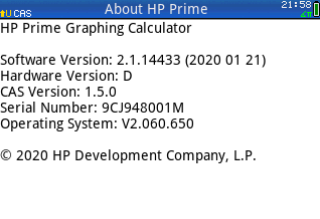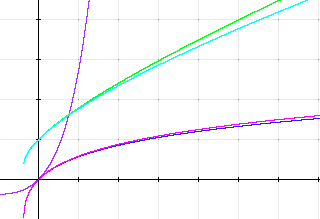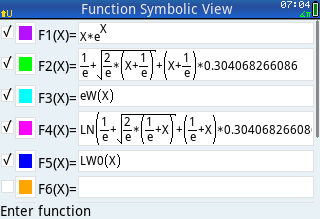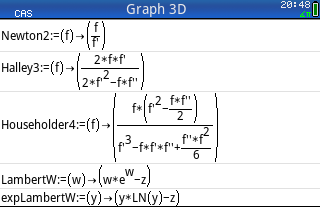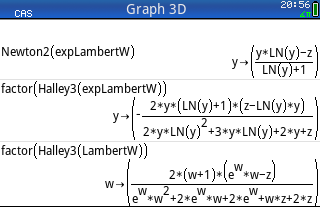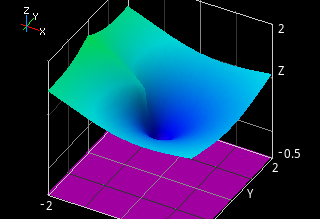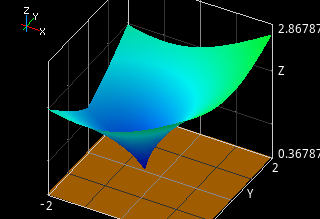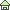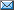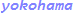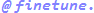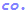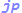© 2000 Takayuki HOSODA.